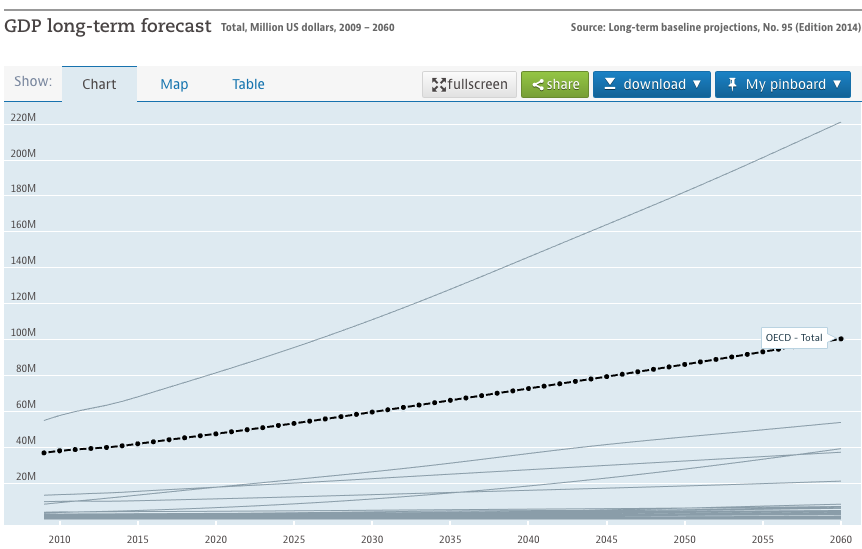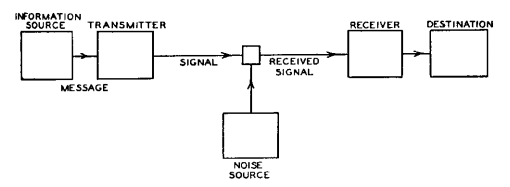# Expected Error, or how wrong you expect to be

3y24th Dec 20168 comments

# 9

Expected value commonly refers to the mean of a distribution that represents the expectations of a future event. It’s much more specific than “mathematical mean”. If one were to ask about the "mean" of a poker hand there would be confusion, but the ‘expected value’ is obvious.

While expected value is a popular term, the fact that it describes one point value means a lot of useful information is excluded.

Say you have two analysts for your Californian flower selling empire. Both hand you forecasts for next year's revenue. One of them tells you that revenue will be between \$8 and \$12 Million, with an average of \$10 million. The other tells you that it will be between -\$50 and \$70 million, with an average of \$10 million. These both have expected values of \$10 million, but I would guess that you would be very interested in the size of those ranges. The uncertainty matters.

One could of course use standard deviation, variance, or literally hundreds of other parameters to describe this uncertainty. But I would propose that these parameters be umbrellaed under the concept of “expected error.” Typically the expected value gets a lot of attention; after all, that is the term in this arena that we have a name for. So an intuitive counter to this focus is the “expected error,” or how much we expect the expected value to be incorrect. In a different sense, the expected error is the part of an estimate that’s not its expected value.

Expected Error: The expected difference between an expected value and an actual value.

Or, “How wrong do you think your best guess will be?”

Hypothetically, any measure of statistical dispersion could be used to describe expected error. Standard deviation, interquartile range, entropy, average absolute deviation, etc. Of these, I think that the mean absolute deviation is probably the most obvious measure to use for expected error when using continuous variables. Expected value uses a mean, so the expected error could be the "expected value" of the error between the actual value and the referenced expected value.

The mean absolute deviation could selectively be divided by the mean to get the mean absolute percentage deviation, in cases where the percentage is more useful than the absolute number. So one could say that a specific forecast has an expected error of “50” or “10%” (in the case of the expected value being 500.)

The most common way to currently describe expected errors is by using margins of error or confidence intervals. These can get much of the point across in many situations but not all. Confidence intervals have difficulties resembling distributions that aren’t very smooth. For instance, say you have a two deals, both with a 98% chance of making \$1 Million. One of them has a 2% chance of making nothing, the other has a 2% chance of losing \$50 Million. A 95% confidence interval would treat these two identically. Mean absolute deviation handles this distinction.

Estimate Comparison

Your two equally-trusted analysts are told to estimate your employee count next year, and return with the expected values of 20 and 43. At this point you can’t really compare them, except for giving each equal weight. With this information alone it’s not very obvious how much they actually agree with each other, or if one spent far longer on the analysis than the other.

Now imagine if the first presented 20 with an expected error of 5, and the other presented 43 with an expected error of 30. Here a story begins to develop. The first figured out some method that made them quite confident. The second wasn’t very sure; a true value of 20 could be reasonable according to the expected error of 30. In this case you’d probably lean closer to 20 than 43.

It’s often fair to say that expected error is negatively correlated with the amount of available information. Say you needed to estimate revenue for 2020. You make a forecast and it has a high expected error. A few years later you have more information, and you make a second forecast with lower expected error. When the time happens you may be able to make a measurement with no expected error.GDP forecast from OECD

For instance, in many graphs of future projections, error bars (proportional to expected error) get larger as time goes further into the future.

This relationship between information and expected error amount does not always hold. One obvious example is a case where one value seems obvious at first, but upon inspection is disproven, leaving several equally-unlikely options available with similar confidence. While new information should always eliminate possible worlds, probability distributions used in expected values act as very specific lenses at those possible worlds.

Propagation of Expected Error

Propagation of error is to propagation of uncertainty what expected value is to mean; it’s somewhat of a specific focus of that concept. The math to understand the propagation of expected error is mostly that for the propagation of uncertainty, but implementation strategy is different.

Most descriptions of the propagation of uncertainty involve understanding how specific margins of errors of inputs correspond to margins of errors of outputs. The mathematics relating inputs to outputs are well understood, so the main question is how to propagate the error through them.

In cases where expected error is calculated, the specific model used to determine an output may be up for consideration. There could be multiple ways to estimate the same output using a set of inputs. In these cases, propagation of expected error can be used as part of the modeling process to determine which ways are the most preferred.

For instance, say you are attempting to estimate the number of piano tuners in Boston. One approach involves an intuitive guess of the number of people who own pianos. A second uses a known number of the piano tuner population in other cities with a linear regression. These approaches will result in different expected values and also different expected errors; we could expect that the expected error of the regression would be much less than that for the much more intuitive and uncertain approach. Discovering this information as part of the modeling process could be used in iterating to find optimal mathematical models.

Expected Error in Communication

In the cases described above expected values and expected values were in reference to specific future outcomes. They could relatedly be applied to one’s expectation of how an agent will understand some communicated information.

Say you consider ‘a few apples’ to be a distribution between 2 to 5. You tell someone else that you have a ‘few apples.’ You probably expect that their definition of ‘few apples’ is likely to have a different distribution than yours. This expected difference between their distribution and yours can be considered the expected error of this aspect of the communication."General communications system" in Communication in the Presence of Noise by Claude E. Shannon

There is a significant study in communication theory about expectations of how noise sources will randomly distort intended signals. In the field of analogue communication and noise, error could slightly change resulting signals according to normal or simple distribution error rates. This is very similar to the concept of expected error, and the concepts of expected error can be used here.

One could imagine an agent making a forecast with expected error E1, then communicating that over a noisy channel with expected error E2, then that information may be misinterpreted with expected error E3. Here it would be useful to treat each expected error term as being represented in similar ways, so that mathematical assumptions could be made to cover the entire pipeline. This may be a bit of a contrived example, but the point is that these different types of error are often handled differently and discussed using very different terminology, and if that could be changed interesting combinations may emerge.

Expected Errors without Expected Values

In communication theory, specific information transferred between two locations isn’t as important as the total noise between the two. Communication systems are optimized to reduce noise without much attention or care about the specific messages transferred. Likewise forecasting and estimation systems could focus on minimizing expected errors.

For instance, someone may be interested in general forecast accuracy, so they may take a survey of the expected errors of a class of estimates of a similar set of complexity within an organization. In other situations they could create grading rubrics or ontologies such to minimize the expected error.

Comparisons to Risk and Uncertainty

The concepts of risk and uncertainty are similar to expected error, so I would like to highlight the differences. First, the terms of risk and uncertainty are both used for many different purposes with slight variations, and have very confusing sets of opposing popular definitions. They both have long histories that tie them to conceptual baggage. As a new term, expected error would have none of that and can be defined separate from expectations.

According to some definitions of risk, risk can be used for both positive and negative outcomes that are uncertain. However, it still strongly implies predictions of future things of consequential impact. If you estimated the number of piano tuners in Boston to be between 5 and 500 for a fun stats problem, I imagine you wouldn’t label that answer as being ‘high risk.’

Uncertainty is closer to the concept but in some cases is awkward. First, it should be mentioned that there is significant literature that assumes that uncertainty is defined as being unquantifiable. Second, in discussions of communication, there is no expected error at the point of a sender, only for for receivers. If uncertainty were to be used it would be have to be understood that it exists isolated to specific agents, which I imagine could be a bit counter-intuitive to some. Perhaps ‘expected error’ can be described as analogues to ‘perspective uncertainty’ or similar narrowed concepts.

Conclusion

While I am reluctant to propose a new term like expected error, I must say that I’ve personally experienced great frustration discussing related concepts without it. In my own thinking, expected error has relevance in such fields as taxonomy, semantics, mathematical modeling, philosophy, and many others.

Thanks to Pepe Swer and Linchuan Zhang for offering feedback on an early draft of this.